• JulieTung/Assignment3

# Assignment 3 Camera Simulation

## Compound Lens Simulator

### Description of implementation approach and comments

After reading the lens data into a vector, I precomputed certain calculations for each lens (the z-index, the zMin and the zMax, etc.) so that they would not have to be computed each time whenever GenerateRay was called. I also computed the ratio by which the raster coordinates and the film coordinates are related (by dividing the given film diagonal (mm) with the diagonal calculated using the film's pixel resolution (pixels).

In GenerateRay, my algorithm works by converting the sample x and y coordinates into film coordinates and then choosing a point on the aperture of the backmost lens as the destination for that ray. I then pass the ray into the lens system, where for each lens, I calculate the refraction. To do this, I have to find each ray-lens intersection, which is done by modeling each lens as a partial sphere. Hence I perform ray-sphere intersection and use a z-min and z-max value in order to ensure that the intersection that I find will actually intersect the aperture of that lens. The ray is then refracted using a form of Snell's law (the vector form that can be found on Wikipedia). For the aperture stop, the effective aperture diameter is the parameter taken from the PBRT file. Here, I just perform a ray-plane intersection to make sure that the ray does not get stopped by the aperture, and I simulate the materials on either side of the aperture to be air (with a refractive index of 1). Any rays that are stopped anywhere along the lens system are given a weight of 0. All other rays have their weights calculated using the formula found in the Kolb paper. The area used is the area of the aperture of the backmost lens, the distance is the length between that aperture and the film plane, and the cosine is found by taking a dot product of the original angle of the ray with the z-axis.

### Final Images Rendered with 512 samples per pixel

 My Implementation Reference Telephoto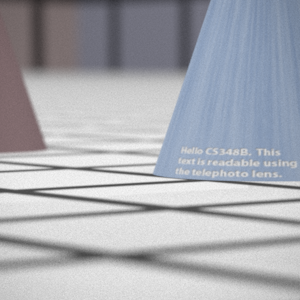Double Gausss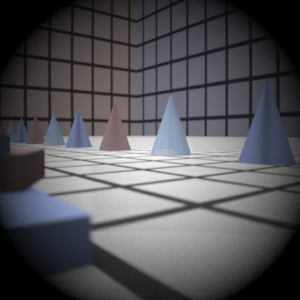Wide Angle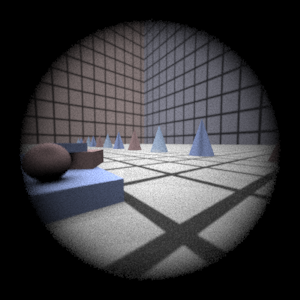Fisheye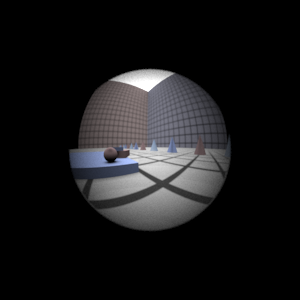### Final Images Rendered with 4 samples per pixel

 My Implementation Reference Telephoto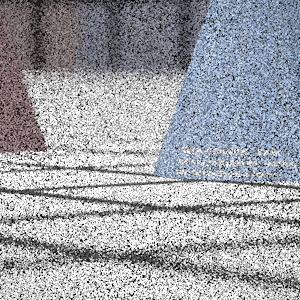Double Gausss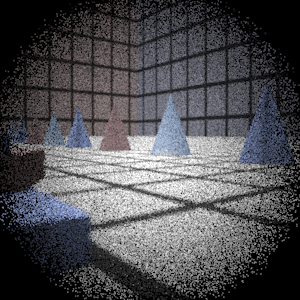Wide Angle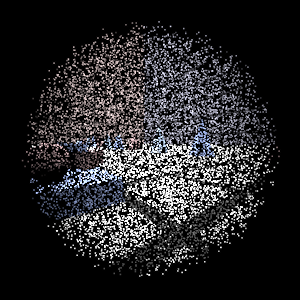Fisheye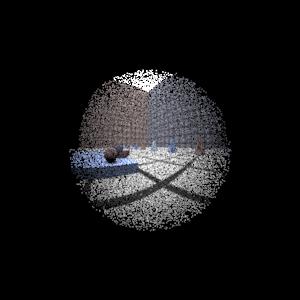## Experiment with Exposure

 Image with aperture full open Image with half radius aperture......

## Autofocus Simulation

......

### Final Images Rendered with 512 samples per pixel

 Adjusted film distance My Implementation Reference Double Gausss 1 mm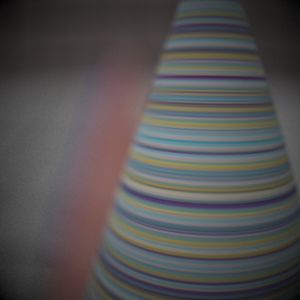Double Gausss 2 mmTelephoto mm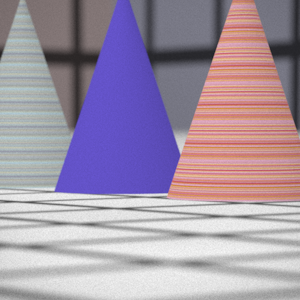## Any Extras

...... Go ahead and drop in any other cool images you created here .....

JulieTung/Assignment3 (last edited 2006-05-09 05:56:54 by JulieTung)